Measure & Add Length (Intro to the Ruler) Video for Kids - Grades K-2
1%
It was processed successfully!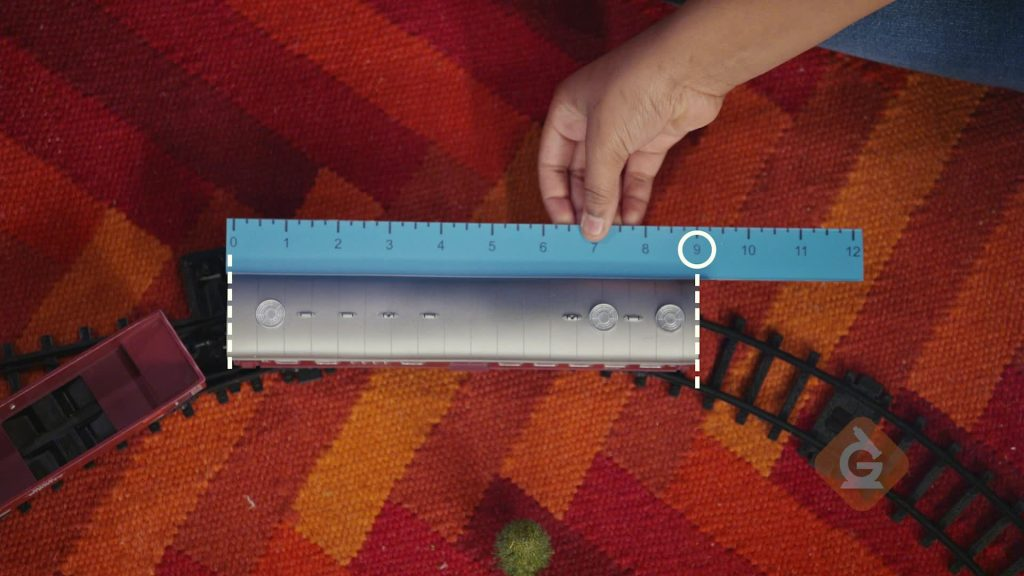HOW DO YOU MEASURE WITH A RULER?

A ruler is a tool you can use to measure how long something is. A ruler has number labels. The first number is 0. You measure the length of an object by lining up one side of your object with the 0. Then see where the other end of the object lines up on the ruler.

To better understand measuring & adding length…

HOW DO YOU MEASURE WITH A RULER?. A ruler is a tool you can use to measure how long something is. A ruler has number labels. The first number is 0. You measure the length of an object by lining up one side of your object with the 0. Then see where the other end of the object lines up on the ruler. To better understand measuring & adding length…

## LET’S BREAK IT DOWN!

### Measure spaceships using paper clips.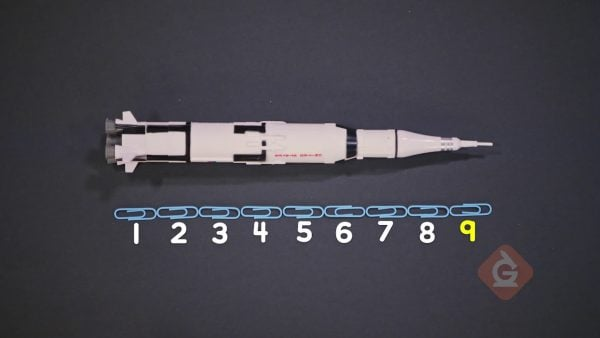You can use everyday objects to measure length. You want to know the length of your toy spaceship. You put one paper clip at the left end of your spaceship. Then you line up more paper clips next to the first and count the number of paper clips from one end to the other. The spaceship is 9 paperclips long.

Measure spaceships using paper clips. You can use everyday objects to measure length. You want to know the length of your toy spaceship. You put one paper clip at the left end of your spaceship. Then you line up more paper clips next to the first and count the number of paper clips from one end to the other. The spaceship is 9 paperclips long.

### Compare the lengths of spaceships.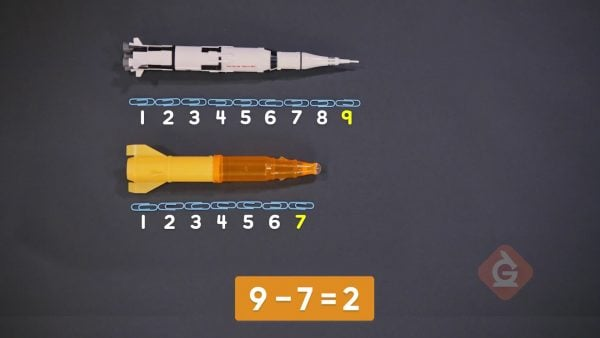Your friend has a toy spaceship that is 7 paper clips long. How much longer is your spaceship than your friend's? 9 – 7 = 2. Your spaceship is 2 paper clips longer than your friend’s.

Compare the lengths of spaceships. Your friend has a toy spaceship that is 7 paper clips long. How much longer is your spaceship than your friend's? 9 – 7 = 2. Your spaceship is 2 paper clips longer than your friend’s.

### Use a ruler to measure toy trains.The numbers on a ruler show equal-sized parts, like your equal-sized paper clips. A ruler is an easier way to measure! This ruler measures in inches. To measure your toy train, you can line up the 0 mark of the ruler at the left end of the train. The right end of the train stops closest to the number 7. The train is 7 inches long.

Use a ruler to measure toy trains. The numbers on a ruler show equal-sized parts, like your equal-sized paper clips. A ruler is an easier way to measure! This ruler measures in inches. To measure your toy train, you can line up the 0 mark of the ruler at the left end of the train. The right end of the train stops closest to the number 7. The train is 7 inches long.

### Use a yardstick to measure toy firetrucks.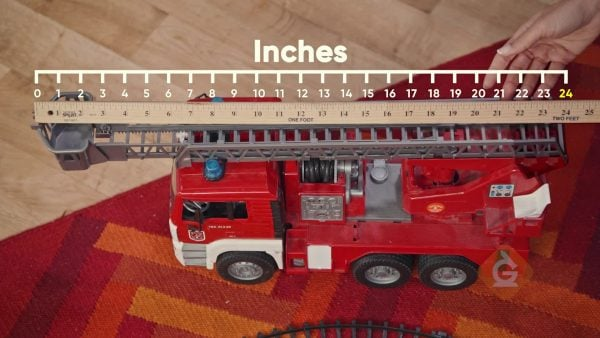A yardstick is a bigger measuring tool. It can measure up to 36 inches. It also has other measurements, like 1 foot. You can use a yardstick to measure your toy firetruck. Line up the 0 mark with the left end of the firetruck. The right end of the firetruck stops near 24 inches. The firetruck is 24 inches long. The yardstick has another label at the same mark, so the firetruck is also 2 feet long.

Use a yardstick to measure toy firetrucks. A yardstick is a bigger measuring tool. It can measure up to 36 inches. It also has other measurements, like 1 foot. You can use a yardstick to measure your toy firetruck. Line up the 0 mark with the left end of the firetruck. The right end of the firetruck stops near 24 inches. The firetruck is 24 inches long. The yardstick has another label at the same mark, so the firetruck is also 2 feet long.

### Measure and compare bridges.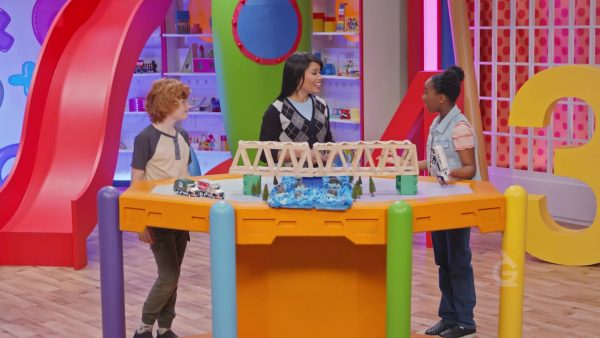You built a bridge that is 20 inches long. Your friend built a bridge that is 16 inches long. How much longer is your bridge? 20 – 16 = 4. Your bridge is 4 inches longer. How long are your bridges together? 20 + 16 = 36. Together, the bridges are 36 inches long.

Measure and compare bridges. You built a bridge that is 20 inches long. Your friend built a bridge that is 16 inches long. How much longer is your bridge? 20 – 16 = 4. Your bridge is 4 inches longer. How long are your bridges together? 20 + 16 = 36. Together, the bridges are 36 inches long.

## MEASURE & ADD LENGTH (INTRO TO THE RULER) VOCABULARY

Length
How long something is from one end to the other end.
Measuring Length
Finding how long something is. You measure length with measuring tools like rulers and yardsticks.
Ruler
A tool used to measure length.
Inch
A unit of measurement that describes length.
Yardstick
A tool used to measure length. It is longer than a ruler.
Foot
A unit of measurement that describes length. It is much bigger than an inch. Each foot equals 12 inches.

## MEASURE & ADD LENGTH (INTRO TO THE RULER) DISCUSSION QUESTIONS

### How can you use paper clips to measure the length of an object?

Use paperclips that are all the same size. Line up the paper clips from one end of the object to the other. Don’t overlap the paper clips or leave any space between them. Count how many paper clips you used. That’s how many paper clips long the object is.

### How can you use a ruler to measure the length of an object?

Line up the zero mark on the ruler with one end of the object. The number next to the other end of the object is its length. If the ruler shows inches, the length is in inches.

### When should you use a yardstick instead of a ruler?

I should use a yardstick to measure objects that are longer than a ruler.

### What are inches and feet? Which is longer: an object that is 1 inch long or an object that is 1 foot long?

Inches and feet are units of measurement that I can use to describe the length of an object. Feet are longer than inches, so an object that is 1 foot long is longer than an object that is 1 inch long.

### Suppose you have two chocolate bars that are different lengths. How can you find how much longer one chocolate bar is than the other? How can you find how long the chocolate bars would be if you combined them end to end?

Measure the lengths of both chocolate bars. Subtract the shorter length from the longer length to find how much longer one chocolate bar is than the other. Add the lengths to find the combined length.
X

## Success

We’ve sent you an email with instructions how to reset your password.
Ok